Examples

Chapter 8 Class 12 Application of Integrals
Serial order wise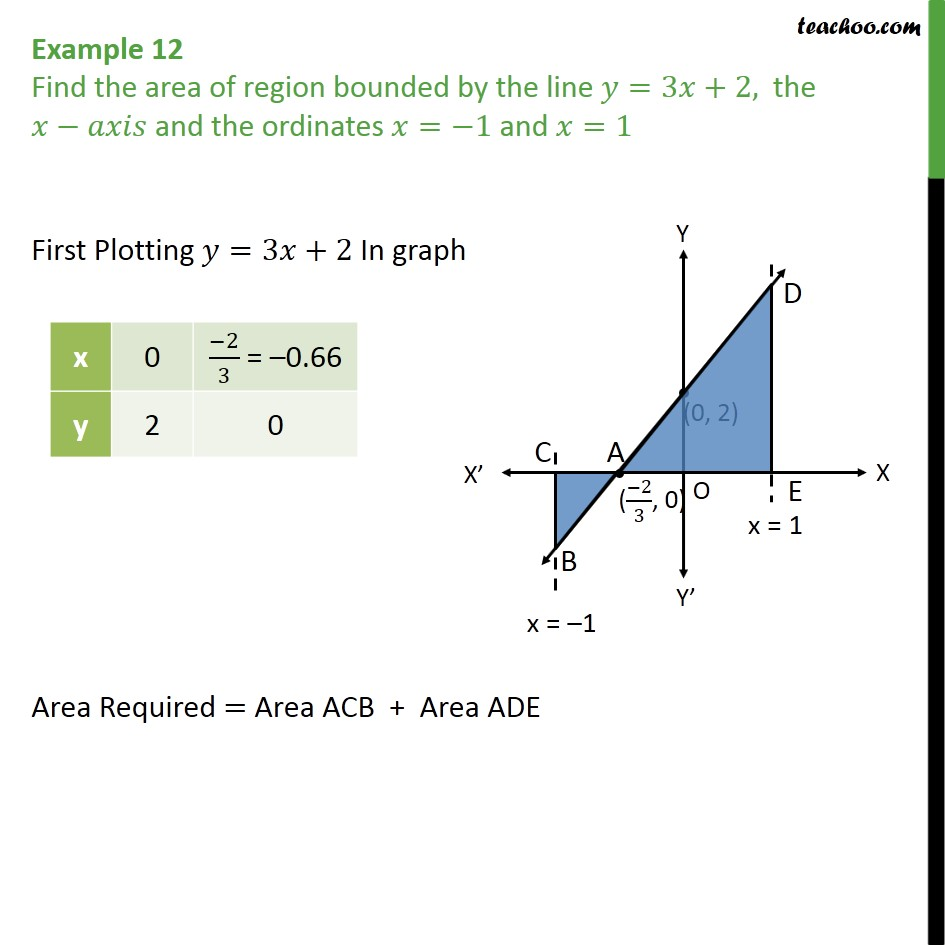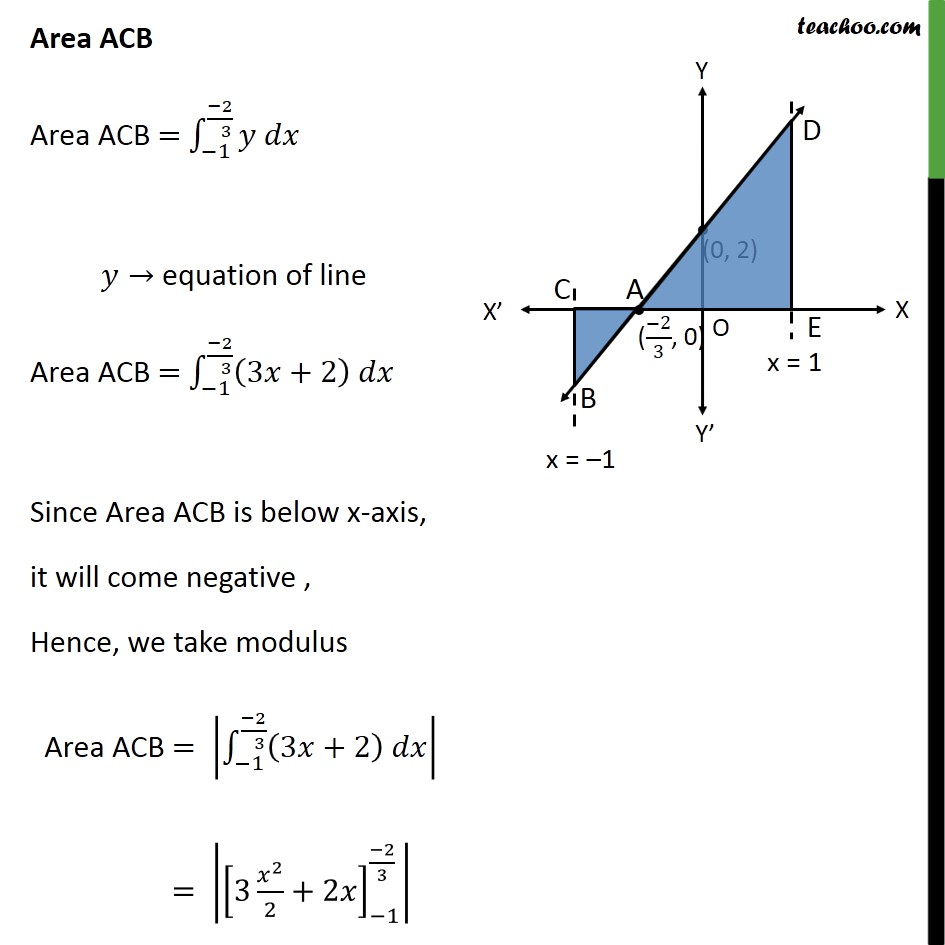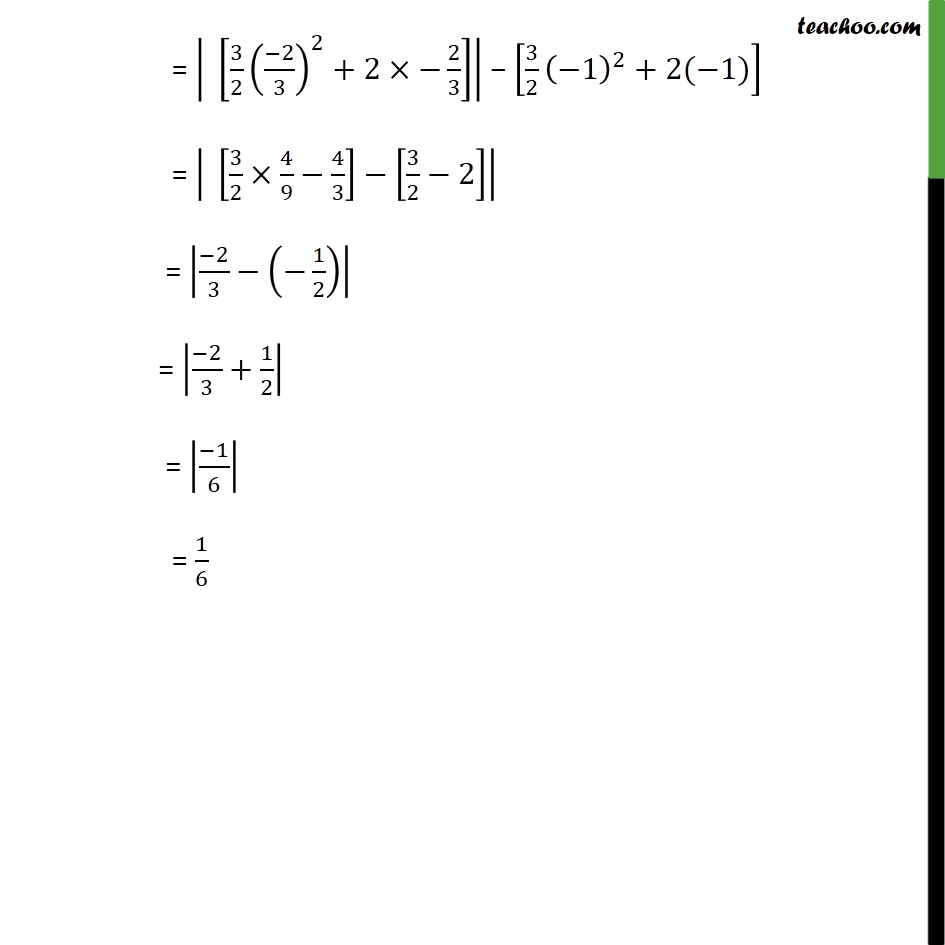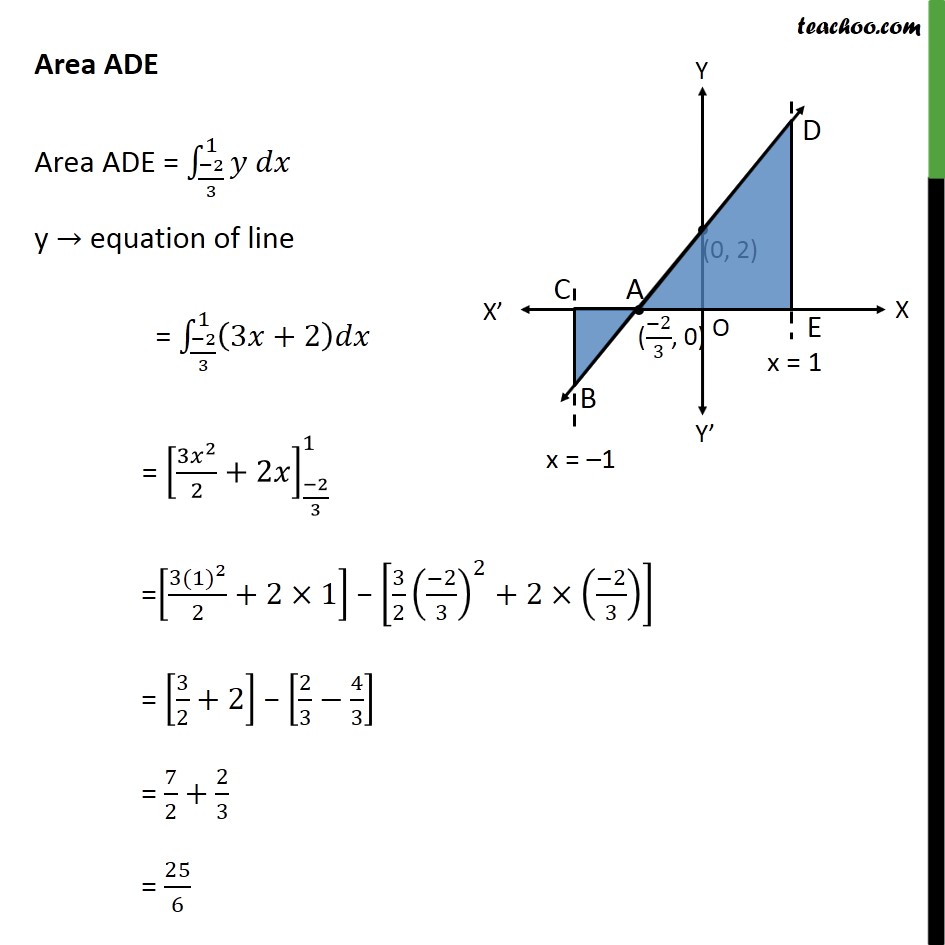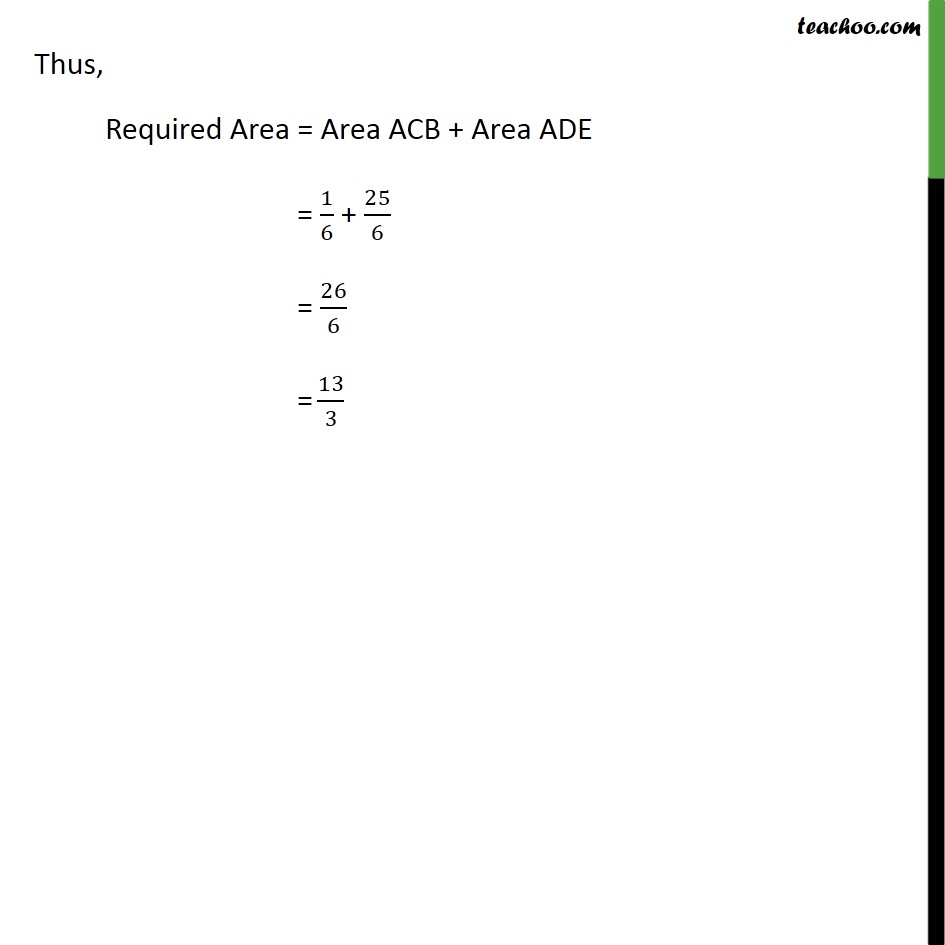Get live Maths 1-on-1 Classs - Class 6 to 12

### Transcript

Example 12 Find the area of region bounded by the line =3 +2, the and the ordinates = 1 and =1 First Plotting =3 +2 In graph Area Required = Area ACB + Area ADE Area ACB Area ACB = 1 2 3 equation of line Area ACB = 1 2 3 3 +2 Since Area ACB is below x-axis, it will come negative , Hence, we take modulus Area ACB = 1 2 3 3 +2 = 3 2 2 +2 1 2 3 = 3 2 2 3 2 +2 2 3 3 2 1 2 +2( 1) = 3 2 4 9 4 3 3 2 2 = 2 3 1 2 = 2 3 + 1 2 = 1 6 = 1 6 Area ADE Area ADE = 2 3 1 y equation of line = 2 3 1 3 +2 = 3 2 2 +2 2 3 1 = 3 (1) 2 2 +2 1 3 2 2 3 2 +2 2 3 = 3 2 +2 2 3 4 3 = 7 2 + 2 3 = 25 6 Thus, Required Area = Area ACB + Area ADE = 1 6 + 25 6 = 26 6 = 13 3